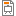# F7ABBPMS - Probability and Mathematical Statistics

Code Completion Credits Range Language
F7ABBPMS Z,ZK 4 2P+2C English
Linear Algebra and Differential Calculus (F7ABBLAD)
Lecturer:
Marek Piorecký (guarantor)
Tutor:
Filip Černý, Marek Piorecký (guarantor), Jan Štrobl
Supervisor:
Department of Biomedical Technology
Synopsis:

Objectives: to familiarize students with the basic principles of the theory of probability and mathematical statistics.

Pre-requisites and entry requirements of the course: Knowledge of mathematics (linear algebra, differential and integral calculus) in the range of F7PBBLAD and F7PBBITP courses taught in the first year of study.

Knowledge, skills, abilities and competencies: The student is acquainted with the probabilistic model, basic definitions of Kolmogorov theory of probability and inductive statistics. The student can apply these definitions to practical problems that arise in other areas of professional work and can explain them sufficiently (e.g. doctors). The student is familiar with the basic methods of inductive statistics and can choose a suitable method for standard statistical problems.

Requirements:

Testimonial/credit:

1) Getting at least 70 % from the total number of points of 3 small tests during the semester (typical examples are shown at the tutorials).

2) Allowed to be absent 3 times during the semester.

3) Active participation in seminars/tutorials, doing homework.

Exam:

Only students that have got testimonial and who have already passed course F7ABBLAD (prerequisite).

Oral exam - 2 open questions on topics / issues from the lectures.

Syllabus of lectures:

1. Motivational lecture. Determinism and randomness.

2. Random variable and its distribution function.

3. Discrete distributions.

4. Continuous distributions.

5. Random vectors, conditioning and independence.

6. Random vectors, characteristics, functions of random variables.

7. The role of mathematical statistics.

8. Parameter estimation. Point estimation of basic characteristics, interval estimation in normal distribution.

9. Methods for construction of point estimations, method of moments, maximum likelihood method. Introduction to Bayesian statistics.

10. Testing hypotheses in normal distribution (one or two selections).

11. Analysis of variance (one way and two way). Testing hypothesis on type of distribution, normality testing.

12. Non-parametric tests.

13. Evaluation of dependence. Correlation and regression analysis.

14. Principles of experimental design.

Syllabus of tutorials:

1. Classical and geometric probability.

2. Combinatorial problems.

3. Discrete variables.

4. Continuous variables.

5. Variable with normal distribution.

6. Conditional and marginal distributions.

7. Bayes' theorem.

8. Point estimation parameters.

9. Interval estimation of parameters.

10. One-sample test of hypothesis.

11. One-sample test of hypothesis about the mean value compared with an interval estimate.

12. Two-sample paired and unpaired test of hypotheses about the mean value.

13. Non-parametric tests.

14. Chi-squared test of hypotheses.

Study Objective:

The objective is to familiarize students with the basic principles of the theory of probability and mathematical statistics.

Study materials:

 CHATFIELD, Christopher. Statistics for technology: a course in applied statistics. 3rd ed. Boca Raton: Chapman &amp; Hall/CRC, 1998. ISBN 0-412-25340-2

 Probability and statistics EBook [online]. USA, University of California, 2005 [cit. 2019-03-16] Last update [2014-03-09]. Available at: http://wiki.stat.ucla.edu/socr/index.php/EBook

 Vladimír Rogalewicz: Pravděpodobnost a statistika pro inženýry, skriptum FBMI, Nakladatelství ČVUT, Praha, 2007 (in Czech).

Note:
The course is a part of the following study plans:

Lectures:
AttachmentSizeLE_2022_1591.66 KBLE_2022_2519.61 KBLE_2022_3529.07 KBLE_2022_4425.95 KBLE_2022_51.22 MBLE_2022_6271.89 KBLE_2002_7668.85 KBLE_2022_8395.43 KBLE_2022_9485.7 KBLE_2022_10297.28 KBLE_2022_111.17 MBLE_2022_12466.92 KBLE_2022_13727.64 KBLecture_01_Introduction_to_the_course_v20200924623.98 KBLecture_07_Principles of statistical methods1.55 MB

Exercises:
AttachmentSize2022 Seminar 1 - Classical and Bayes probability108.33 KB2022 Seminar 1 - Results116.5 KB2022 Seminar 2 - Discrete random variable196.86 KB2022 Seminar 2 - Results260.78 KB2022 Seminar 3 - Discrete random vector638.19 KB2022 Seminar 3 - Results205.47 KB2022 Seminar 4 - Continuous random variable, continuous random vector172.76 KB2022 Seminar 4 - Results241.43 KB2022 Seminar 5 - Special continuous distributions127.7 KB2022 Seminar 5 - Results183.5 KB2022 Seminar 6+7 - Point and interval estimations, Wilcoxon's non-parametrical test162.32 KB2022 Seminar 6+7 - Results225.35 KB2022 Seminar 8 - One-sample parametrical statistic tests425.38 KB2022 Seminar 8 - Results171.91 KB2022 Seminar 9 - Tests of normality, nonparametric test583.59 KB2022 Seminar 9 - Results307.68 KB2022 Seminar 10 - Two sample tests236.28 KB2022 Seminar 10 - Results1.08 MB2022 Seminar 11 - Paired two sample tests115.86 KB2022 Seminar 11 - Results196.97 KB2022 Seminar 12 - Multiple sample tests442.87 KB2022 Seminar 12 - Results222.9 KBHartley's F-Max test - table93.48 KBF test table183.16 KBMann Whitney test94.87 KBTabulated values of selected distributions -From doc. Rogalewicz scripta416.71 KBTables_from_the_script_of_assoc_prof_Rogalewicz_v201811051.73 MBGUIDEBOOK FOR STUDENTS OF (BIO)-STATISTICS801.2 KBExamTopicsSuggestions_v2019101442.67 KB